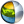Cg 3.1 Toolkit Documentation Cg / Standard Library / sincos

## Name

sincos - returns sine of scalars and vectors.

## Synopsis

```void sincos(float a, out float s, out float c);
void sincos(float1 a, out float1 s, out float1 c);
void sincos(float2 a, out float2 s, out float2 c);
void sincos(float3 a, out float3 s, out float3 c);
void sincos(float4 a, out float4 s, out float4 c);

void sincos(half a, out half s, out half c);
void sincos(half1 a, out half1 s, out half1 c);
void sincos(half2 a, out half2 s, out half2 c);
void sincos(half3 a, out half3 s, out half3 c);
void sincos(half4 a, out half4 s, out half4 c);

void sincos(fixed a, out fixed s, out fixed c);
void sincos(fixed1 a, out fixed1 s, out fixed1 c);
void sincos(fixed2 a, out fixed2 s, out fixed2 c);
void sincos(fixed3 a, out fixed3 s, out fixed3 c);
void sincos(fixed4 a, out fixed4 s, out fixed4 c);
```

## Parameters

a
Input vector or scalar of which to determine the sine and cosine.
s
Ouput vector or scalar for sine results.
c
Ouput vector or scalar for cosine results.

## Description

Outputs to s the sine of a in radians, and outputs to c the cosine of a in radians. The output values are in the range [-1,+1].

For vectors, the output vectors contains the sine or cosine respectively of each element of the input vector.

## Reference Implementation

sin is best implemented as a native sine instruction, however sin for a float scalar could be implemented by an approximation like this.

```void sincos(float3 a, out float3 s, float3 out c)
{
int i;

for (i=0; i<3; i++) {
s[i] = sin(a[i]);
c[i] = cos(a[i]);
}
}
```

## Profile Support

sincos is fully supported in all profiles unless otherwise specified.

sincos is supported via an approximation (shown above) in the vs_1_1, vp20, and arbvp1 profiles.

sincos is unsupported in the fp20, ps_1_1, ps_1_2, and ps_1_3 profiles.| Cg Toolkit | Download | Release Archive | Profiles | Reference | Books | Discussions |